## Beta diversity measures

The following beta diversity measures are available:

• Bray-Curtis: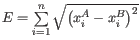• Jaccard: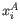• Euclidean:where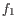is the number of OTUs andandare the abundances of OTU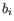in samples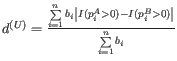and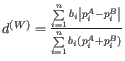, respectively.

The following distances are also available:

• Unweighted UniFrac: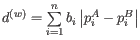• Weighted UniFrac: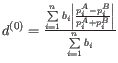• Weighted UniFrac not normalized: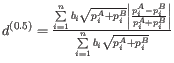• D_0 UniFrac: The generalized UniFrac distance• D_0.5 UniFrac: The generalized UniFrac distancewhereis the number of branches in the phylogenetic tree,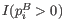is the length of branch;and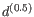are the proportion of taxa descending from branchfor samplesand, respectively; and the indicator functions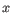and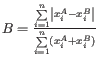assume the value of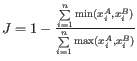if any taxa descending from branchis present in samplesand, respectively, or 0 otherwise.

The unweighted UniFrac distance gives comparatively more importance to rare lineages, while the weighted UniFrac distance gives more important to abundant lineages. The generalized UniFrac distance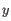offers a robust tradeoff [Chen et al., 2012].